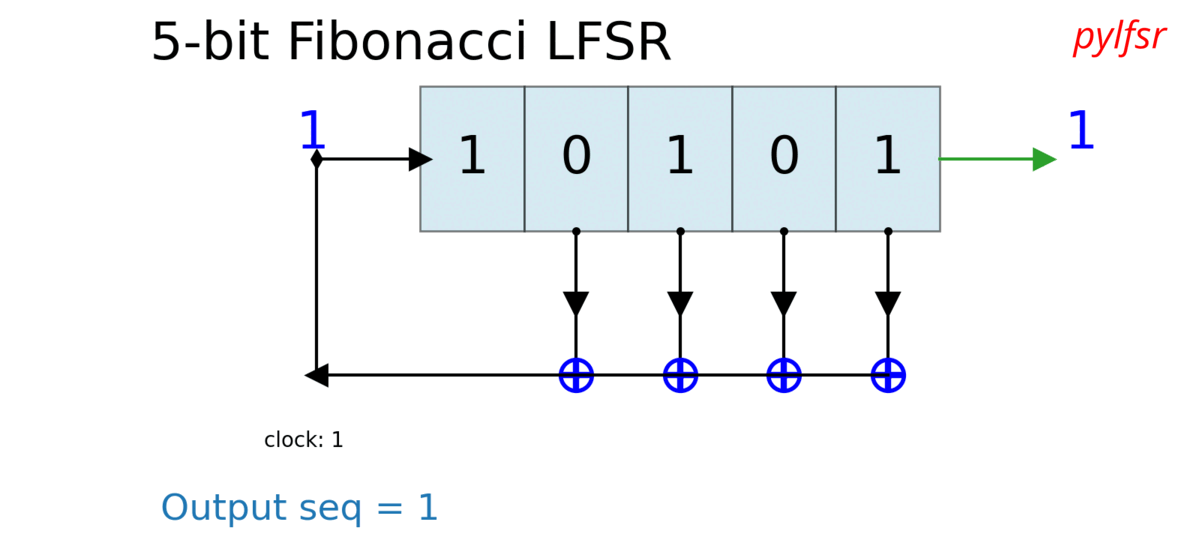# PyLFSR: Linear Feedback Shift Register¶

### Github Page | Discussion Forum | GithubLinear Feedback Shift Register - Documentation.

PyLFSR is an open source Python library to create Pseudo-Random Generator based on Linear Feedback Shift Registe. It is easy to use and create Pseudo-Random Generator, with custom configurations, such as A5/1, its enhacement, Geffe Genrator and many more. A generated sequence can be tested across different properties.

A simple example of generating 5-bit LFSR with feedback polynomial of p(x) = x5+ x3+1

```import numpy as np
from pylfsr import LFSR

state = [0,0,0,1,0]
fpoly = [5,3]
L = LFSR(fpoly=fpoly,initstate =state)

# Generate K-bits
k=10
seq_k  = L.runKCycle(k)

print('10 bits')
print(L.arr2str(seq_k))
print('')

# Generate bits of one full period.
# Expected period of LFSR = 2^M-1, for M-bit LFSR).
seq_full  = L.runFullPeriod()

print('Full period of LFSR = 31-cycles')
print(L.arr2str(seq_full))

# Vizualise LFSR.
L.Viz()
```
```10 bits
0100001001

Full period of LFSR = 31-cycles
01000010010110011111000110111010100001001
```Authors/Contacts:

Feedback/Contact/Contribute

• n.bajaj[AT]qmul.ac.uk

• n.bajaj[AT]imperial.ac.uk

• nikkeshbajaj[AT]gmail[dot?]

Nikesh Bajaj: http://nikeshbajaj.in

Queen Mary University of London

Imperial Collage London